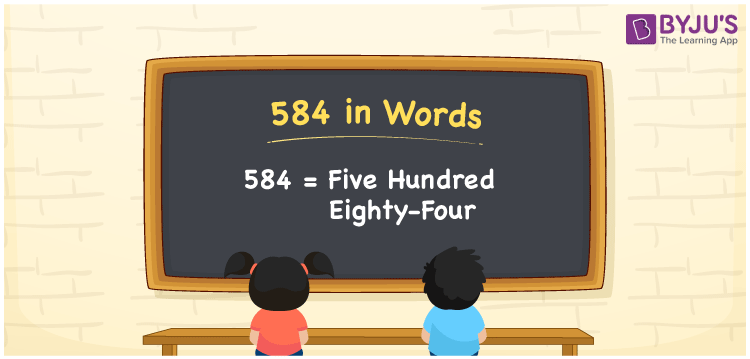# 584 in Words

584 in words is written as Five hundred eighty-four. In both the International System of Numerals and the Indian System of Numerals, 584 is written as Five hundred eighty-four. The number 584 is a Cardinal Number as it represents some quantity. For example, the “cost of the jacket is rupees 584”.

 584 in Words Five hundred eighty-four Five hundred eighty-four in Number 584

## 584 in English Words

We write 584 in English Words using the letters of the English alphabet. Therefore, we read 584 in English as “Five hundred eighty-four”.## How to Write 584 in Words?

To write 584 in words, we shall use the place value chart. In the place value chart, write 5 in the hundreds, 8 in the tens, and 4 in the ones, respectively. Now let us make a place value chart to write the number 584 in words.

 Hundreds Tens Ones 5 8 4

Thus, we can write the expanded form as

5 × Hundred + 8 × Ten + 4 × One

= 5 × 100 + 8 × 10 + 4 × 1

= 500 + 80 + 4

= 584

= Five hundred eighty-four.

584 is a natural number, the successor of 583 and the predecessor of 585.

584 in words – Five hundred eighty-four

• Is 584 an odd number? – No
• Is 584 an even number? – Yes
• Is 584 a perfect square number? – No
• Is 584 a perfect cube number? – No
• Is 584 a prime number? – No
• Is 584 a composite number? – Yes

## Frequently Asked Questions on 584 in Words

Q1

### How to write 584 in words?

584 in words is written as Five hundred eighty-four.
Q2

### How to write 584 in the International and Indian System of Numerals?

In both, the system of numerals, 584 in words, is written as Five hundred eighty-four.
Q3

### What is the successor of 584?

The successor of 584 is 585.